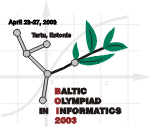Baltic Olympiad in Informatics
April 23-27, 2003, Tartu, Estonia

# Table (task with open input)

For the given integer M, build a square table with N rows and N columns (2 ≤ N ≤ 10), filled with decimal digits, with the following restriction: the N-digit numbers formed by the digits in each table row (from left to right), each table column (from top to bottom) and each main diagonal (from top to bottom) must be multiples of M, must not start with the digit 0 and must be unique within the table.

For example, a valid table for M = 2 is

``` 2 3 4
5 6 6
8 2 0
```

The following tables are not valid for M = 2:

``` 4
```

because N < 2;

``` 2 0
4 8
```

because the numbers in the last column and on one of the main diagonals start with the digit 0;

``` 2 3 4
5 8 8
2 0 2
```

because the number 482 is present twice in the table.

It is not always possible to solve this task. For example, the task is unsolvable for M = 10.

## Input data

You are given ten test files TABLEx.IN (1 ≤ x ≤ 10), each containing one value of M.

## Output data

You must find a valid table for each test case and write it into the corresponding output file TABLEx.OUT (1 ≤ x ≤ 10). The first line of a file must contain N, the number of rows and columns in the table. The i+1-st line of the file (1 ≤ i ≤ N) must contain the elements of the i-th row of the table as N digits, separated by spaces.

## Sample

 TABLE.IN TABLE.OUT 2 3 2 3 4 5 6 6 8 2 0

## Remark

It is known that there will be at least one solution for each given test input.